Main Content

# Motorized Camera - Multi-Input Multi-Output Nonlinear ARX and Hammerstein-Wiener Models

This example shows how to estimate multi-input multi-output (MIMO) nonlinear black box models from data. Two types of nonlinear black box models are offered in the System Identification Toolbox™ - Nonlinear ARX and Hammerstein-Wiener models.

### The Measured Data Set

The data saved in the file motorizedcamera.mat will be used in this example. It contains 188 data samples, collected from a motorized camera with a sample time of 0.02 seconds. The input vector u(t) is composed of 6 variables: the 3 translation velocity components in the orthogonal X-Y-Z coordinate system fixed to the camera [m/s], and the 3 rotation velocity components around the X-Y-Z axes [rad/s]. The output vector y(t) contains 2 variables: the position (in pixels) of a point which is the image taken by the camera of a fixed point in the 3D space. We create an IDDATA object `z` to hold the loaded data: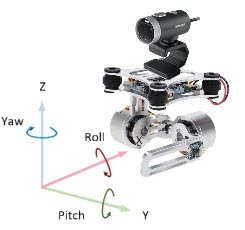```load motorizedcamera z = iddata(y, u, 0.02, 'Name', 'Motorized Camera', 'TimeUnit', 's'); ```

### Nonlinear ARX (IDNLARX) Model - Picking Regressors

Let us first try nonlinear ARX models. Two important elements need to be chosen: the model regressors and the nonlinear mapping functions.

The regressors are simple formulas based on time-delayed I/O variables, the simplest case being the variables lagged by a small set of consecutive values. For example, if "u" in the name of the input variable, and "y" the name of the output variables, then an example regressor set can be { y(t-1), y(t-2), u(t), u(t-1), u(t-2) }, where "t" denotes the time variable. Another example involving polynomial terms could be {y(t-2)^4, y(t-2)*u(t-1), u(t-4)^2}. More complex, arbitrary formulas in the delayed variables are also possible.

The easiest way of specifying linear regressors is by using an "orders matrix". This matrix takes the form NN = [na nb nk] and it specifies by how many lags each output (na) and each input variable (nb, nk) are delayed. This is the same idea that is used when estimating the linear ARX models (see ARX, IDPOLY). For example, NN = [2 3 4] implies the regressor set {y(t-2), u(t-4), u(t-5), u(t-6)}. In the general case of models with NY outputs and NU inputs, NN is a matrix with NY rows and NY+2*NU rows.

### Nonlinear ARX (IDNLARX) Model - Preliminary Estimation Using Wavenet

To start, let us choose the orders NN = [na nb nk] = [ones(2,2), ones(2,6), ones(2,6)]. It means that each output variable is predicted by all the output and input variables, each being delayed by 1 sample. The model equation can be written as y_i(t) = F_i(y1(t-1), y2(t-1), u1(t-1), u2(t-1), u3(t-1)), i = 1, 2. Let us choose a Wavelet Network (wavenet) as the nonlinear mapping function for both outputs. The function NLARX estimates the parameters of the Nonlinear ARX model.

```NN = [ones(2,2), ones(2,6), ones(2,6)]; % the orders mw1 = nlarx(z, NN, wavenet); ```

Examine the result by comparing the output simulated with the estimated model and the output in the measured data `z`:

```compare(z,mw1) ```### Nonlinear ARX Model - Trying Higher Orders

Let us check if we can do better with higher orders. Note that when identifying models using basis function expansions to express the nonlinearity, the number of model parameters can exceed the number of data samples. In such cases, some estimation metrics such as Noise Variance and Final Prediction Error (FPE) cannot be determined reliably. For the current example, we turn off the warning informing us about this limitation.

```ws = warning('off','Ident:estimation:NparGTNsamp'); % mw2 = nlarx(z, [ones(2,2), 2*ones(2,6), ones(2,6)], wavenet) compare(z,mw2) ```
```mw2 = <strong>Nonlinear ARX model with 2 outputs and 6 inputs</strong> Inputs: u1, u2, u3, u4, u5, u6 Outputs: y1, y2 Regressors: Linear regressors in variables y1, y2, u1, u2, u3, u4, u5, u6 Output functions: Output 1: Wavelet Network with 27 units Output 2: Wavelet Network with 25 units Sample time: 0.02 seconds Status: Estimated using NLARX on time domain data "Motorized Camera". Fit to estimation data: [99.22;99.15]% (prediction focus) FPE: 0.1262, MSE: 0.4453 ```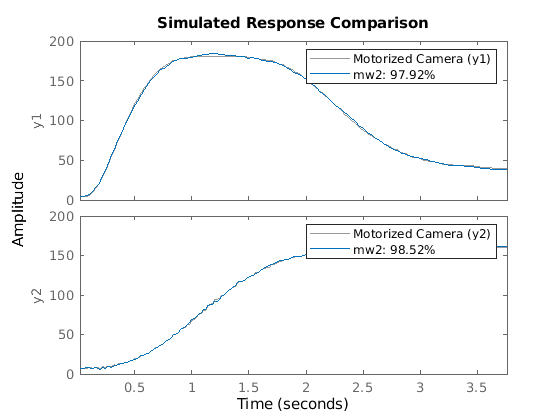The second model mw2 is pretty good. So let us keep this choice of model orders in the following examples.

```nanbnk = [ones(2,2), 2*ones(2,6), ones(2,6)]; % final orders ```

To view the regressor set implied by this choice of orders, use the GETREG command:

```getreg(mw2) ```
```ans = 14×1 cell array {'y1(t-1)'} {'y2(t-1)'} {'u1(t-1)'} {'u1(t-2)'} {'u2(t-1)'} {'u2(t-2)'} {'u3(t-1)'} {'u3(t-2)'} {'u4(t-1)'} {'u4(t-2)'} {'u5(t-1)'} {'u5(t-2)'} {'u6(t-1)'} {'u6(t-2)'} ```

The numbers of units ("wavelets") of the two WAVENET function have been automatically chosen by the estimation algorithm. These numbers are displayed below.

```mw2.OutputFcn(1).NonlinearFcn.NumberOfUnits ```
```ans = 27 ```

### Nonlinear ARX Model - Adjusting Number of Units of Nonlinear Functions

The number of units in the WAVENET function can be explicitly specified instead of being automatically chosen by the estimation algorithm. Suppose we want to use 10 units for the first nonlinear mapping function, and 5 for the second one (note that the model for each output uses its own mapping function; the array of all mapping functions is stored in the model's "OutputFcn" property).

```Fcn1 = wavenet(10); % output function for the first output Fcn2 = wavenet(5); % output function for the second output mw3 = nlarx(z, nanbnk, [Fcn1; Fcn2]) ```
```mw3 = <strong>Nonlinear ARX model with 2 outputs and 6 inputs</strong> Inputs: u1, u2, u3, u4, u5, u6 Outputs: y1, y2 Regressors: Linear regressors in variables y1, y2, u1, u2, u3, u4, u5, u6 Output functions: Output 1: Wavelet Network with 10 units Output 2: Wavelet Network with 5 units Sample time: 0.02 seconds Status: Estimated using NLARX on time domain data "Motorized Camera". Fit to estimation data: [99.01;98.89]% (prediction focus) FPE: 0.2273, MSE: 0.7443 ```

### Nonlinear ARX Model - Trying Other Nonlinear Mapping Functions

In place of the WAVENET function, other nonlinear functions can also be used. Let us try the TREEPARTITION for both outputs.

```mt1 = nlarx(z, nanbnk, 'treepartition'); ```

In the above call, we have used the string 'treepartition' in place of an object to specify the mapping function. This syntax facilitates a quick way of constructing the model with the limitation that all the mapping functions must be of the same type, and they must be used with their default property values. In the following example, the treepartition object constructor is called to directly create a Tree-partition nonlinear function object.

```mt2 = nlarx(z, nanbnk, treepartition) ```
```mt2 = <strong>Nonlinear ARX model with 2 outputs and 6 inputs</strong> Inputs: u1, u2, u3, u4, u5, u6 Outputs: y1, y2 Regressors: Linear regressors in variables y1, y2, u1, u2, u3, u4, u5, u6 Output functions: Output 1: Tree Partition with 7 units Output 2: Tree Partition with 7 units Sample time: 0.02 seconds Status: Estimated using NLARX on time domain data "Motorized Camera". Fit to estimation data: [98.56;98.34]% (prediction focus) MSE: 1.615 ```

Similarly, we can use a Sigmoid Network (SIGMOIDNET) as the mapping function. Estimation options such as maximum iterations (MaxIterations) and iteration display can be specified using the `nlarxOptions` command.

```opt = nlarxOptions('Display','on'); opt.SearchOptions.MaxIterations = 5; ms1 = nlarx(z, nanbnk, 'sigmoidnet', opt); ```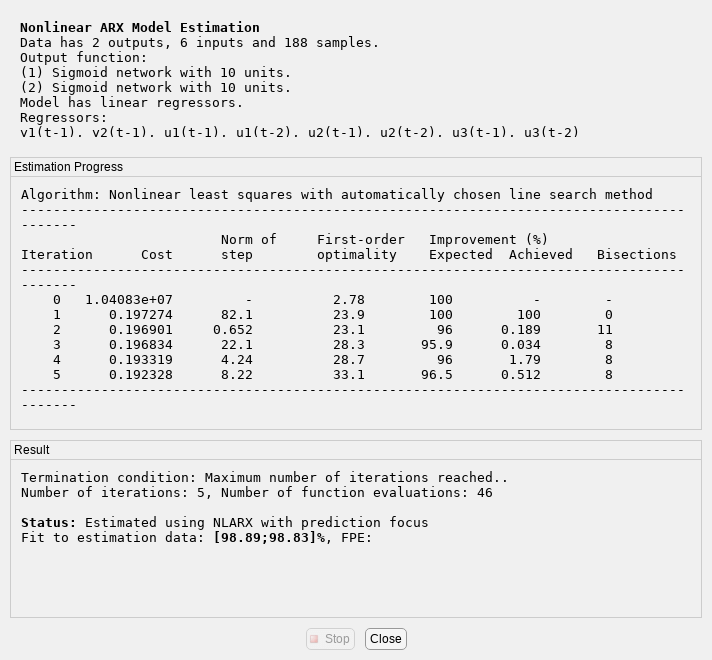This calling syntax for NLARX is very similar to the ones used before - specifying the data, the orders and the nonlinear mapping functions as their primary input arguments. However, to modify the estimation options from their default values, we constructed an option set, `opt`, using the nlarxOptions command and passed it to the NLARX command as an additional input argument.

### Nonlinear ARX Model with Mixed Nonlinear Functions

It is possible to use different nonlinear functions on different output channels in the same model. Suppose we want to use a tree partition function to describe the first output and use a wavelet network for the second output. The model estimation is shown below. The third input argument (the nonlinear mapping function) is now an array of two different functions.

```Fcn1 = treepartition; Fcn2 = wavenet; mtw = nlarx(z, nanbnk, [Fcn1; Fcn2]); ```

Sometimes the function that maps the regressors to the model output need not be nonlinear; it could simply be a weighted sum of the regressor vectors, plus an optional offset. This is similar to the linear ARX models (except for the offset term). The absence of nonlinearity in an output channel can be indicated by choosing a LINEAR function. The following example means that, in model_output(t) = F(y(t-1), u(t-1), u(t-2)), the function F is composed of a linear component for the first output, and a nonlinear component (SIGMOIDNET) for the second output.

```Fcn1 = linear; Fcn2 = sigmoidnet(2); opt.Display = 'off'; % do not show estimation progress anymore mls = nlarx(z, nanbnk, [Fcn1; Fcn2], opt) ```
```mls = <strong>Nonlinear ARX model with 2 outputs and 6 inputs</strong> Inputs: u1, u2, u3, u4, u5, u6 Outputs: y1, y2 Regressors: Linear regressors in variables y1, y2, u1, u2, u3, u4, u5, u6 Output functions: Output 1: Linear Function Output 2: Sigmoid Network with 2 units Sample time: 0.02 seconds Status: Estimated using NLARX on time domain data "Motorized Camera". Fit to estimation data: [98.72;98.79]% (prediction focus) FPE: 0.5594, MSE: 1.05 ```

There is no nonlinearity in the model for the first output. It is not, however, purely linear because of the presence of an offset term.

```disp(mls.OutputFcn(1)) ```
```<strong>Linear Function</strong> Inputs: y1(t-1), y2(t-1), u1(t-1), u1(t-2), u2(t-1), u2(t-2), u3(t-1), u3(t-2), u4(t-1), u4(t-2), u5(t-1), u5(t-2), u6(t-1), u6(t-2) Output: y1 Nonlinear Function: None Linear Function: initialized to [48.3 -32.2 -0.229 -0.0705 -0.113 -0.0516 -0.182 0.297 0.199 -0.133 -0.337 0.583 -0.448 0.167] Output Offset: initialized to 109 Input: [1×1 idpack.Channel] Output: [1×1 idpack.Channel] LinearFcn: [1×1 nlident.internal.ProjectedLinearFcn] Offset: [1×1 nlident.internal.Offset] ```

We have the option of making it purely linear by choosing to not use the offset term. This choice can be made by fixing the offset to a zero value before estimation.

```Fcn1.Offset.Value = 0; Fcn1.Offset.Free = false; mlsNoOffset = nlarx(z, nanbnk, [Fcn1; Fcn2], opt); ```

### Inspection of Estimation Results

Various models can be compared in the same COMPARE command.

```compare(z, mw2, ms1, mls) ```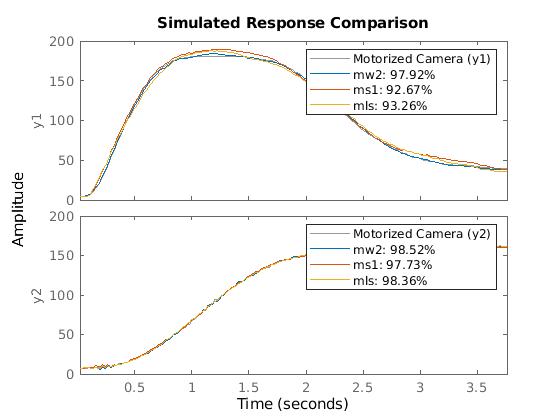Function PLOT may be used to view the nonlinearity responses of various models.

```plot(mt1,mtw,ms1,mls) ```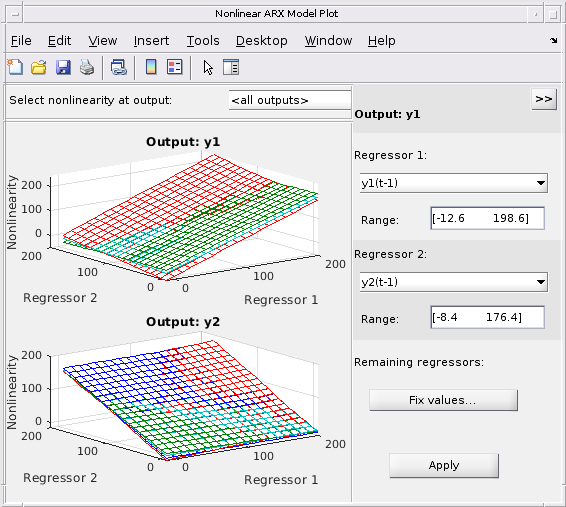Note that the control panel on the right hand side of the plot allows for regressor selection and configuration.

Other functions such as RESID, PREDICT and PE may be used on the estimated models in the same way as in the case of linear models.

### Hammerstein-Wiener (IDNLHW) Model - Preliminary Estimation

A Hammerstein-Wiener model is composed of up to 3 blocks: a linear transfer function block is preceded by a nonlinear static block and/or followed by another nonlinear static block. It is called a Wiener model if the first nonlinear static block is absent, and a Hammerstein model if the second nonlinear static block is absent.

IDNLHW models are typically estimated using the syntax:

```M = NLHW(Data, Orders, InputNonlinearity, OutputNonlinearity). ```

where Orders = [nb nf nk] specifies the orders and delay of the linear transfer function. InputNonlinearity and OutputNonlinearity specify the nonlinear functions for the two nonlinear blocks. The linear output error (OE) model corresponds to the case of trivial (UNITGAIN) nonlinearities.

### Estimation of Hammerstein Model (No Output Nonlinearity)

Let us choose Orders = [nb bf nk] = [ones(2,6), ones(2,6), ones(2,6)]. It means that, in the linear block, each output is the sum of 6 first order transfer functions driven by the 6 inputs. Try a Hammerstein model (no output nonlinearity) with the input nonlinearity described by a piecewise linear function. Notice that the entered single PWLINEAR object is automatically expanded to all the 6 input channels. UNITGAIN indicates absence of nonlinearity.

```opt = nlhwOptions(); opt.SearchOptions.MaxIterations = 15; NN = [ones(2,6), ones(2,6), ones(2,6)]; InputNL = pwlinear; % input nonlinearity OutputNL = unitgain; % output nonlinearity mhw1 = nlhw(z, NN, InputNL, OutputNL, opt) ```
```mhw1 = Hammerstein-Wiener model with 2 outputs and 6 inputs Linear transfer function matrix corresponding to the orders: nb = [1 1 1 1 1 1; 1 1 1 1 1 1] nf = [1 1 1 1 1 1; 1 1 1 1 1 1] nk = [1 1 1 1 1 1; 1 1 1 1 1 1] Input nonlinearities: Input 1: Piecewise Linear Input 2: Piecewise Linear Input 3: Piecewise Linear Input 4: Piecewise Linear Input 5: Piecewise Linear Input 6: Piecewise Linear Output nonlinearities: Output 1: absent Output 2: absent Sample time: 0.02 seconds Status: Estimated using NLHW on time domain data "Motorized Camera". Fit to estimation data: [98.46;97.93]% FPE: 7.928, MSE: 2.216 ```

Examine the result with COMPARE.

```compare(z, mhw1); ```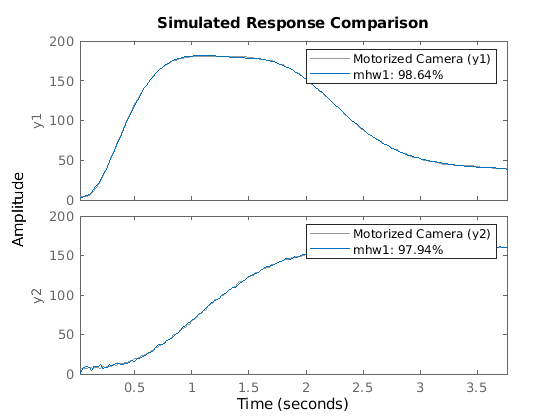Model properties can be visualized by the PLOT command.

Click on the block-diagram to choose the view of the input nonlinearity (UNL), the linear block or the output nonlinearity (YNL).

When the linear block view is chosen, by default all the 12 channels are shown in very reduced sizes. Choose one of the channels to have a better view. It is possible to choose the type of plots within Step response, Bode plot, Impulse response and Pole-zero map.

```plot(mhw1) ```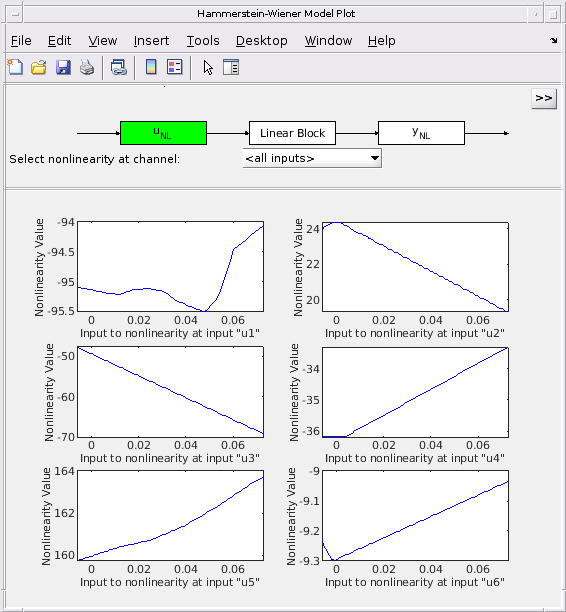The result shown by COMPARE was quite good, so let us keep the same model orders in the following trials.

```nbnfnk = [ones(2,6), ones(2,6), ones(2,6)]; ```

### Estimation of Wiener Model (No Input Nonlinearity)

Let us try a Wiener model. Notice that the absence of input nonlinearity can be indicated by "[]" instead of the UNITGAIN object.

```opt.SearchOptions.MaxIterations = 10; mhw2 = nlhw(z, nbnfnk, [], 'pwlinear', opt) ```
```mhw2 = Hammerstein-Wiener model with 2 outputs and 6 inputs Linear transfer function matrix corresponding to the orders: nb = [1 1 1 1 1 1; 1 1 1 1 1 1] nf = [1 1 1 1 1 1; 1 1 1 1 1 1] nk = [1 1 1 1 1 1; 1 1 1 1 1 1] Input nonlinearities: Input 1: absent Input 2: absent Input 3: absent Input 4: absent Input 5: absent Input 6: absent Output nonlinearities: Output 1: Piecewise Linear Output 2: Piecewise Linear Sample time: 0.02 seconds Status: Estimated using NLHW on time domain data "Motorized Camera". Fit to estimation data: [73.85;71.36]% FPE: 1.314e+05, MSE: 503.8 ```

### Estimation of Hammerstein-Wiener Model (Both Input and Output Nonlinearities)

Indicate both input and output nonlinearities for a Hammerstein-Wiener model. As in the case of Nonlinear ARX models, we can use a character vector (rather than object) to specify the nonlinear mapping functions.

```mhw3 = nlhw(z, nbnfnk,'saturation', 'pwlinear', opt) ```
```mhw3 = Hammerstein-Wiener model with 2 outputs and 6 inputs Linear transfer function matrix corresponding to the orders: nb = [1 1 1 1 1 1; 1 1 1 1 1 1] nf = [1 1 1 1 1 1; 1 1 1 1 1 1] nk = [1 1 1 1 1 1; 1 1 1 1 1 1] Input nonlinearities: Input 1: Saturation Input 2: Saturation Input 3: Saturation Input 4: Saturation Input 5: Saturation Input 6: Saturation Output nonlinearities: Output 1: Piecewise Linear Output 2: Piecewise Linear Sample time: 0.02 seconds Status: Estimated using NLHW on time domain data "Motorized Camera". Fit to estimation data: [86.88;84.55]% FPE: 1.111e+04, MSE: 137.3 ```

The limit values of the SATURATION function can be accessed as follows:

```mhw3.InputNonlinearity(1).LinearInterval % view Linear Interval of SATURATION ```
```ans = 0.0103 0.0562 ```

Similarly, the break points of the PWLINEAR function can be accessed as follows:

```mhw3.OutputNonlinearity(1).BreakPoints ```
```ans = Columns 1 through 7 17.1233 34.2491 51.3726 68.4968 85.6230 102.7478 119.8742 2.6184 16.0645 45.5178 41.9621 62.3246 84.9038 112.2970 Columns 8 through 10 136.9991 154.1238 171.2472 135.4543 156.1016 173.2701 ```

### Hammerstein-Wiener Model - Using Mixed Nonlinear Functions

Different nonlinear functions can be mixed in a same model. Suppose we want a model with: - No nonlinearity on any output channels ("Hammerstein Model") - Piecewise linear nonlinearity on input channel #1 with 3 units - Saturation nonlinearity on input channel #2 - Dead Zone nonlinearity on input channel #3 - Sigmoid Network nonlinearity on input channel #4 - No nonlinearity (specified by a unitgain object) on input channel #5 - Sigmoid Network nonlinearity on input channel #6 with 5 units

We can create an array of nonlinear mapping function objects of chosen types and pass it to the estimation function NLHW as input nonlinearity.

```inputNL = [pwlinear; saturation; deadzone; sigmoidnet; unitgain; sigmoidnet(5)]; inputNL(1).NumberOfUnits = 3; opt.SearchOptions.MaxIterations = 25; mhw4 = nlhw(z, nbnfnk, inputNL, [], opt); % "[]" for 4th input denotes no output nonlinearity ```

### Hammerstein-Wiener Model - Specifying Initial Guess for SATURATION and DEADZONE

The initial guess for the linear interval of SATURATION and the zero interval of DEADZONE can be directly indicated when these objects are created; you can also specify constraints on these values such as whether they are fixed to their specified values (by setting Free attribute to false), or if their estimations are subject to minimum/maximum bounds (using the Minimum and Maximum attributes).

Suppose we want to set the saturation's linear interval to [10 200] and the deadzone's zero interval to [11 12] as initial guesses. Furthermore, we want the upper limit of the saturation to remain fixed. We can achieve this configuration as follows.

```% Create nonlinear functions with initial guesses for properties. OutputNL1 = saturation([10 200]); OutputNL1.Free(2) = false; % the upper limit is a fixed value OutputNL2 = deadzone([11 12]); mhw5 = idnlhw(nbnfnk, [], [OutputNL1; OutputNL2], 'Ts',z.Ts); ```

Notice the use of the IDNLHW model object constructor `idnlhw`, not the estimator `nlhw`. The resulting model object `mhw5` is not yet estimated from data. The limit values of the saturation and deadzone functions can be accessed. They still have their initial values, because they are not yet estimated from data.

```mhw5.OutputNonlinearity(1).LinearInterval % show linear interval on saturation at first output channel after estimation mhw5.OutputNonlinearity(2).ZeroInterval % show zero interval on dead zone at second output channel after estimation ```
```ans = 10 200 ans = 11 12 ```

What are the values of these limits after estimation?

```opt.SearchOptions.MaxIterations = 15; mhw5 = nlhw(z, mhw5, opt) % estimate the model from data mhw5.OutputNonlinearity(1).LinearInterval % show linear interval on saturation at first output channel after estimation mhw5.OutputNonlinearity(2).ZeroInterval % show zero interval on dead zone at second output channel after estimation ```
```mhw5 = Hammerstein-Wiener model with 2 outputs and 6 inputs Linear transfer function matrix corresponding to the orders: nb = [1 1 1 1 1 1; 1 1 1 1 1 1] nf = [1 1 1 1 1 1; 1 1 1 1 1 1] nk = [1 1 1 1 1 1; 1 1 1 1 1 1] Input nonlinearities: Input 1: absent Input 2: absent Input 3: absent Input 4: absent Input 5: absent Input 6: absent Output nonlinearities: Output 1: Saturation Output 2: Dead Zone Sample time: 0.02 seconds Status: Estimated using NLHW on time domain data "Motorized Camera". Fit to estimation data: [27.12;6.857]% FPE: 3.373e+06, MSE: 4666 ans = 9.9974 200.0000 ans = 11.0020 12.0011 ```

### Post Estimation Analysis - Comparing Different Models

Models of different nature (IDNLARX and IDNLHW) can be compared in the same COMPARE command.

```compare(z,mw2,mhw1) warning(ws) % reset the warning state ```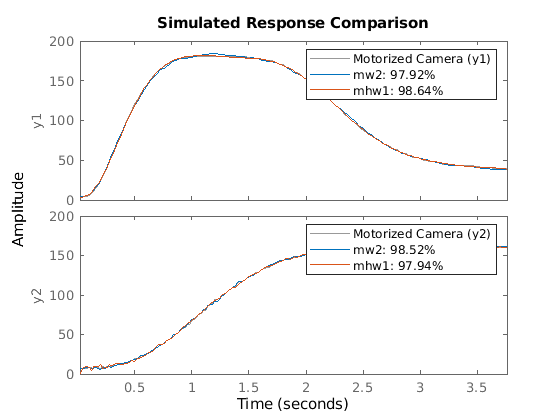## SupportGet trial now